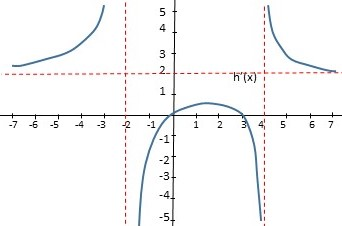# Write an equation for the function graphed below.

## Question:

Write an equation for the function graphed below.## Rational Functions

Rational Functions are functions of the form:

{eq}h(x) = \frac{ f(x) }{ g(x) } {/eq}

Where f(x) and g(x) are polynomials.

The rational function has vertical asymptotes, ( vertical lines that the curve of the function approaches more and more as x approaches some value x=a) at the zeros of the denominator function g(x).

Also, the rational function has horizontal asymptotes (horizontal lines that the curve approaches more and more as x goes to infinity or negative infinity) if and only if both the numerator f(x) and the denominator g(x) have the same degree.

First, we know the function must be a rational function because it has vertical and horizontal asymptotes. So, {eq}h (x) = \frac {f(x)}{g(x)} {/eq}

...

Become a Study.com member to unlock this answer! Create your account